#### This is number Phi

This is the visual interpretation of Phi - a Golden ratio number, which is 0.618033988749894848204586... To see a Golden ratio description, click here. The grid on this webpage represents decimal digits of number Phi: starting at top left corner each line distance is a next decimal digit. The precision of number used here is 1000 digits.

Use the sliders at the top of the page to zoom onto decimals. To see the decimals in correspondence to pixels in ratio 1:1 click here, or set the first slider to a very minimum and point your cursor to the top left corner of the webpage. To enjoy the interaction fun, move the cursor across the wabpage and try also this preset 1 and preset 2, or try a random values.

This webpage was created by

CLOSE

# Golden ratio

is a ratio of circa 62 to 38.
It occures in nature frequently.

To be precise, Golden ratio is
1.618033988749894848204586834365638117720309179805762862135448622705260462818902449707207204189391137484754088075386891752126633862223536931793180060766726354433389086595939582905638322661319928290267880675208766892501711696207032221043216269548626296313614438149758701220340805887954454749246185695364864449241044320771344947049565846788509874339442212544877066478091588460749988712400765217057517978....
...and it continues up to infinty. In following examples we will use just value 1.62 or 1.618.

It’s commonly denoted as Greek letter Phi (φ).

## Basic definition## Algebraic form## Why value 1.618 ?

If you want to get following image, where it is stated that ratio of inside rectangles is preserved ...... thus following spiral, the Golden spiral ...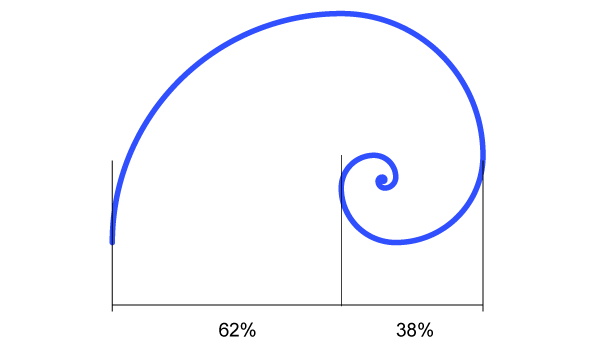... draw an equilateral square, ...... and multiply the width using such value “X”, so that on the right we will get a rectangle with the same width to height ratio as parent / original / covering rectangle: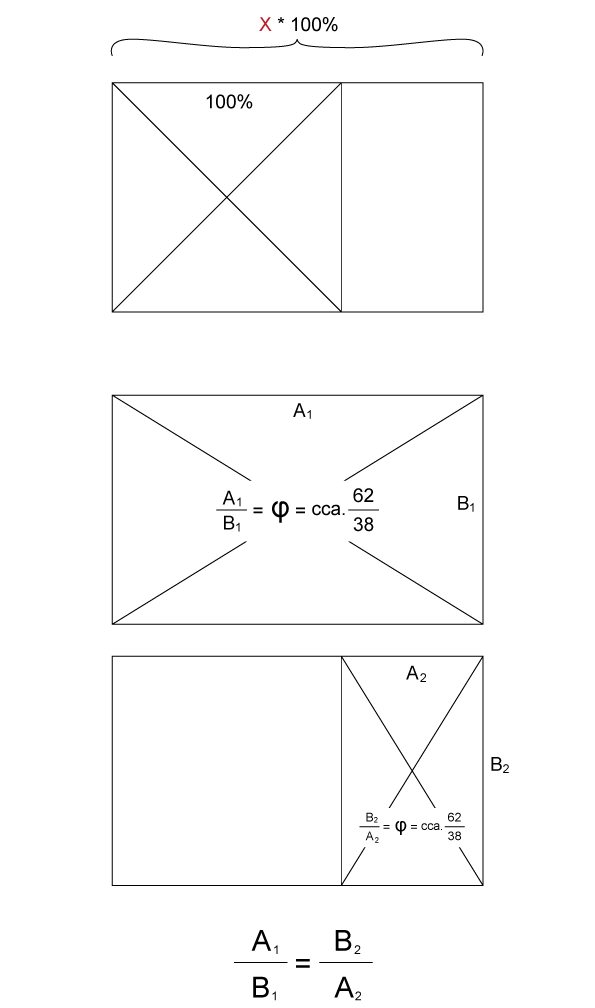The searched value “X” is 1.618033988...., the Golden ratio, cca. 1.6.

Then, we can continue to do the same procedure - to draw an equilateral square inside the space left, ...... and the space left this time will be in the same ratio as two parent / previous / containing rectangles.After repeatring the same procedure of inscribibg squares, at the end, you will get following picture:where the Golden spiral appears:This spiral is special, because the ratio repeats in it.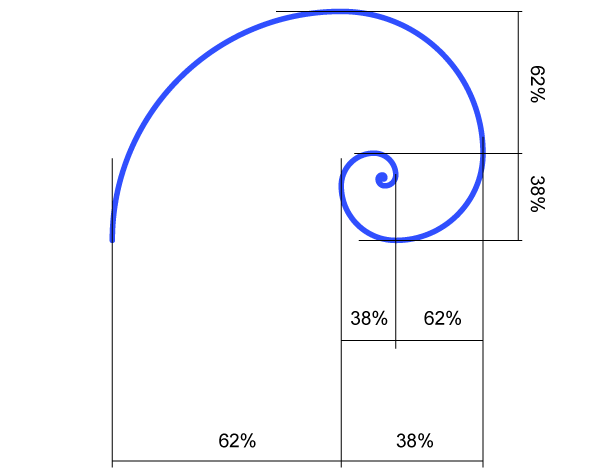It’s a logarithmic spiral whose growth factor is cca. 1.618, the Golden ratio.

## Another magic with 1.618

If we draw a line with a length of Golden ratio 1.618 and mark on it value 1, ...... and mark the line using percentages, ...... the found percentage value X is 61.8033....% and the number covers exactly the same digits as are the digits those we can find in Golden ratio 1.618033....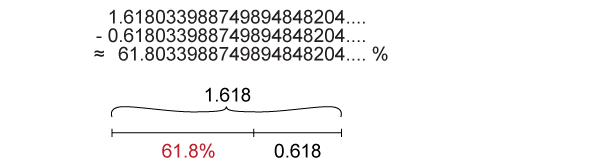## Golden ratio is an interesting number, becauseGolden ratio with 50,000 decimals:
http://www2.cs.arizona.edu/icon/oddsends/phi.htm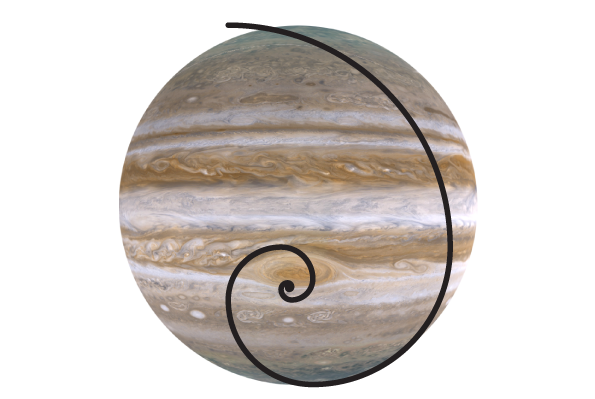An example of Golden ratio
using the Great Red Spot (the eye) of Jupiter.

## Notice, that Fibonacci numbers

0, 1, 1, 2, 3, 5, 8, 13, 21, 34, 55, 89, 144, ... (each number is the sum of the two preceding ones) could be interpreted using Golden ratio too: each side length is a successive Fibonacci number. Fibonacci sequence appear in nature frequently.